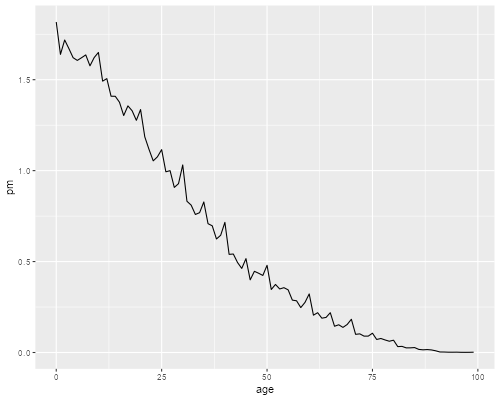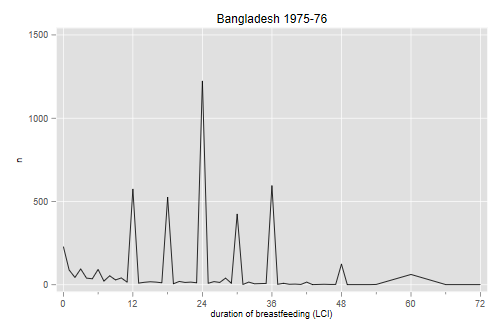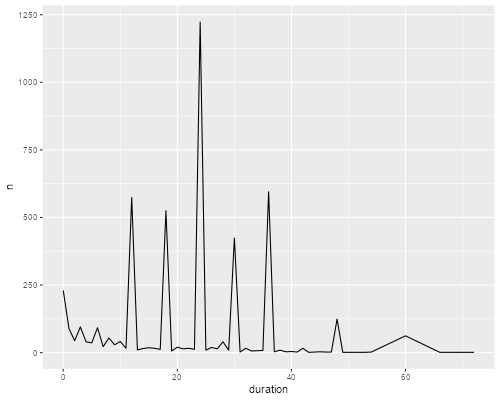Germán Rodríguez
Demographic Methods Princeton University## Digit Preference

The datasets section has the age distribution of the Philippines in 1990 from ages 0 to 99, which appears as table 7.7 in Siegel and Swanson (2004), p. 103. (Thanks to Tom Pullum for providing an electronic version as well as a do file to analyze it.) We’ll calculate some measures of digit preference using Stata and R.

We first read the data and plot the age distribution in single years of age.

```. infile age pop using ///
>     https://grodri.github.io/datasets/phpop1990.dat, clear

. gen pm = pop/1000000

. line pm age, ytitle("Population (millions)") ///
>   title(Philippines 1990)

. graph export phpop1990.png, replace width(500)
file phpop1990.png saved as PNG format
``````> library(dplyr)
> library(ggplot2)
+   header = FALSE, col.names = c("age", "pop")) |>
+   mutate(pm = pop/1000000)
> ggplot(ph, aes(age, pm)) + geom_line()
> ggsave("phpop1990r.png", width=500/72, height=400/72, dpi=72)
```The spikes are likely to represent some form of age misreporting. To investigate a preference for terminal digits such as 0 or 5 we could tabulate the last digit of age:

```. gen lastdigit = mod(age,10)

. tab lastdigit [fw=pop]

lastdigit │      Freq.     Percent        Cum.
────────────┼───────────────────────────────────
0 │  7,611,712       12.57       12.57
1 │  6,374,857       10.53       23.10
2 │  6,424,913       10.61       33.71
3 │  6,042,858        9.98       43.69
4 │  6,003,475        9.91       53.60
5 │  6,140,517       10.14       63.74
6 │  5,546,993        9.16       72.90
7 │  5,664,798        9.35       82.25
8 │  5,344,036        8.82       91.08
9 │  5,401,935        8.92      100.00
────────────┼───────────────────────────────────
Total │ 60,556,094      100.00
```
```> ph <- mutate(ph, last.digit = age %% 10)
> total <- sum(ph\$pop)
> ph |>  group_by(last.digit) |>
+   summarize(freq = sum(pop), percent = 100*freq/total)
# A tibble: 10 × 3
last.digit    freq percent
<dbl>   <int>   <dbl>
1          0 7611712   12.6
2          1 6374857   10.5
3          2 6424913   10.6
4          3 6042858    9.98
5          4 6003475    9.91
6          5 6140517   10.1
7          6 5546993    9.16
8          7 5664798    9.35
9          8 5344036    8.82
10          9 5401935    8.92
```

We see a strong preference for 0, and some for 1, 2 and 5. Note, however, that because of mortality we should expect more people at age 0 than 5 or 9, and more at 10 than 15 or 19, and so forth, with more at 80 than 85 or 89.

Myers’ adjustment is to tabulate the last digit several times starting at different values. In our example we could tabulate it only for ages 0 to 89, and then 1 to 90, until we do 9 to 98, and sum the tabulations, obtaining a “blended” population which helps control for the trend.

This is equivalent to counting all ages 10 times, except ages 0 to 8 (which are counted 1 to 9 times, respectively) and 90 to 98 (which are counted 9 to 1 times, respectively). So we can obtain the result more easily using a weight:

```. gen mw = 10

. replace mw = age+1 if age < 9

. replace mw = 99-age if age > 89

. replace mw = 0 if age > 98

. gen combow = pop*mw

. tab lastdigit [fw=combow], matcell(blended)

lastdigit │      Freq.     Percent        Cum.
────────────┼───────────────────────────────────
0 │ 59,752,360       11.28       11.28
1 │ 50,629,836        9.56       20.84
2 │ 52,212,367        9.86       30.70
3 │ 50,395,096        9.51       40.21
4 │ 51,921,770        9.80       50.01
5 │ 54,969,894       10.38       60.39
6 │ 50,600,297        9.55       69.94
7 │ 53,367,794       10.07       80.02
8 │ 51,854,354        9.79       89.81
9 │ 54,002,900       10.19      100.00
────────────┼───────────────────────────────────
Total │529,706,668      100.00
```
```> phb <- mutate(ph, mw = ifelse(age < 9, age+1, ifelse(age > 89, 99-age, 10)))
> totalb <- sum(phb\$pop * phb\$mw)
> blended <- phb |> group_by(last.digit) |>
+   summarize(freq = sum(pop*mw), pct = 100*freq/totalb)
```

Now that we have the counts we need to divide by the sum and see how far we are from 10% in each digit. This is best done with Mata

```: b = st_matrix("blended")

: f = 100 * b :/ sum(b)

: sum( abs(f :- 10) )/2
1.927534882
```
```> sum(abs(blended\$pct - 10))/2
 1.927535
```

So we would need to reclasify almost 2 percent of the cases. The blended frequencies show some preference for 0, but no marked preference for 5.

### A Stata Command

I have wrapped these calculations in a Stata command called `myers`. To install it in net-aware Stata type `net from https://grodri.github.io/demography`, and follow the instructions. The command has online help, which you should consult to learn about the options, which include `range(bot top)`, to specify the (initial) range of ages to be tabulated, and `months`, for studying heaping in duration data. To reproduce the results above try `myers age[fw=pop], range(0 89)`. The command stores the index in `r(myers)`.

### An R Function

I have wrapped these calculations in an R function called `myers()`. You can get the source code using `source("https://grodri.github.io/demography/myers.R")` The function signature is `myers(data, age, weight, limits=NULL, months=FALSE)`. (The argument `months` is used to study heaping in duration data.) To reproduce the results above try `myers(ph, age, pop, c(0, 89))` I also provide a `print()` method that displays the estimate, a `summary()` method that prints the table of blended frequencies, and a `plot()` method that displays the deviations.

The data analyzed above are used as an example in Siegel and Swanson (2004). They state that they cover the range 10-89, but in fact they continue the sum all the way to 99 (excluding 100, which is left out of our dataset). This is equivalent to assuming that the frequencies above 99 are all zero. We can reproduce their results exactly using `myers age [fw=pop], range(10 99)``myers(ph, age, pop, c(10, 99))`.

Here are reported durations of breastfeeding in the last closed interval (the interval between the next-to-last and last child) from the Bangladesh WFS:

```. infile duration n using ///
>   https://grodri.github.io/datasets/bdblci.dat, clear

. twoway (line n duration) , title(Bangladesh 1975-76) ///
>   xtitle("duration of breastfeeding (LCI)") ///
>   xlabel( 0(12)72 ) xmtick(6(12)66) legend(off)

. graph export bdblci.png, width(500) replace
file bdblci.png saved as PNG format
``````> bd <- read.table("https://grodri.github.io/datasets/bdblci.dat",
+   header = FALSE, col.names = c("duration", "n"))
> ggplot(bd, aes(duration, n)) + geom_line()
> ggsave("bdblcir.png", width=500/72, height=400/72, dpi=72)
```It seems quite clear that there is a marked preference for multiples of a year as well as multiples of 6 months. It is possible, of course, that women will aim to wean the child at age one, two, or three. Later on we will see from current status evidence that this is not likely to be the case.

We can calculate Myers’ index, using the months option, and specifying a range of 0 to 59, which spans 60 months, an exact multiple of 12, and leaves (at least) 11 more months of data for blending.

```. myers duration [fw=n], range(0,59) months

Last digit │      Freq.     Percent        Cum.
────────────┼───────────────────────────────────
0 │     31,104       62.59       62.59
1 │        454        0.91       63.50
2 │        648        1.30       64.81
3 │        800        1.61       66.42
4 │        932        1.88       68.29
5 │        504        1.01       69.31
6 │     12,253       24.66       93.97
7 │        284        0.57       94.54
8 │        942        1.90       96.43
9 │        566        1.14       97.57
10 │        751        1.51       99.08
11 │        456        0.92      100.00
────────────┼───────────────────────────────────
Total │     49,694      100.00

Myers' Blended Index = 70.581291
```
```> source("https://grodri.github.io/demography/myers.R")
> m <- myers(bd, duration, n, c(0, 59), TRUE)
> summary(m)

Myers's blended frequencies
digit  freq        pct
1      0 31104 62.5910573
2      1   454  0.9135912
3      2   648  1.3039804
4      3   800  1.6098523
5      4   932  1.8754779
6      5   504  1.0142069
7      6 12253 24.6569002
8      7   284  0.5714976
9      8   942  1.8956011
10     9   566  1.1389705
11    10   751  1.5112488
12    11   456  0.9176158

Myers' blended index = 70.58
```

So, 63% of the cases report an exact multiple of 12 and another 25% report a multiple of 6 (but not 12). We would need to reclassify 71% of the cases to obtain a uniform distribution by month.

This dataset does not exhibit a marked trend through the range the way an age distribution may do, so you would expect very similar results by just dividing duration by 12 and tabulating the remainder. We just need to make sure we give each month an equal chance by going up to 59 or 71 months. Try

```. gen dmod12 = mod(dur,12)

. tab dmod12 [fw=n] if dur < 60 // or < 72

dmod12 │      Freq.     Percent        Cum.
────────────┼───────────────────────────────────
0 │      2,746       60.03       60.03
1 │        112        2.45       62.48
2 │         87        1.90       64.39
3 │        130        2.84       67.23
4 │        101        2.21       69.44
5 │         60        1.31       70.75
6 │      1,059       23.15       93.90
7 │         31        0.68       94.58
8 │         92        2.01       96.59
9 │         52        1.14       97.73
10 │         66        1.44       99.17
11 │         38        0.83      100.00
────────────┼───────────────────────────────────
Total │      4,574      100.00
```
```> filter(bd, duration < 60) |>
+   group_by(remainder = duration %% 12) |>
+   summarize(freq = sum(n)) |>
+   mutate(pct = round(100*freq/sum(freq),2) )
# A tibble: 12 × 3
remainder  freq   pct
<dbl> <int> <dbl>
1         0  2746 60.0
2         1   112  2.45
3         2    87  1.9
4         3   130  2.84
5         4   101  2.21
6         5    60  1.31
7         6  1059 23.2
8         7    31  0.68
9         8    92  2.01
10         9    52  1.14
11        10    66  1.44
12        11    38  0.83
```

The relative frequencies are very similar to the blended ones.

